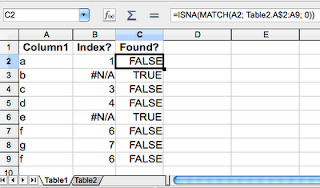## Sunday, April 10, 2011

### Two Useful Excel FunctionsI often use Excel as a tool for some tests or data analysis. For example, last week I tried to comparing two columns in data base to see their difference. I found out that I had an Excel as a tool to do that. This file contains some formula for column comparison. Here is summary what I did for my future reference.

First, I copied column from table 1 to a worksheet(table1), and another column from table 2 to another worksheet(table2). Then I added a formula like this in column B:

`=MATCH(A2, Table1!A2:A2409, 0)`

MATCH function is useful to find first parameter value from the second parameter array, and the third parameter 0 is used to find a match. The result will be the index in the array if item is found, or #NA if nothing is found.

The result will be index number or #NA. I prefer to view the result as true or false. ISNA() is a function for this purpose.above are snapshots of NeoOffice at my iMac.